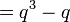# Group cohomology of projective general linear group of degree two over a finite field

## Contents

This article gives specific information, namely, group cohomology, about a family of groups, namely: projective general linear group of degree two. This article restricts attention to the case where the underlying ring is a finite field.
View group cohomology of group families | View other specific information about projective general linear group of degree two | View other specific information about group families for rings of the type finite field

## Particular cases$q$ (field size)$p$ (underlying prime, field characteristic) Case for$q$ Group$PGL(2,q)$ Order of the group ($= q^3 - q$) Group cohomology page
2 2 even symmetric group:S3 6 group cohomology of symmetric group:S3
3 3 odd symmetric group:S4 24 group cohomology of symmetric group:S4
4 2 even alternating group:A5 60 group cohomology of alternating group:A5
5 5 odd symmetric group:S5 120 group cohomology of symmetric group:S5
7 7 odd projective general linear group:PGL(2,7) 336 group cohomology of projective general linear group:PGL(2,7)
8 2 even projective special linear group:PSL(2,8) 504 group cohomology of projective special linear group:PSL(2,8)
9 3 odd projective general linear group:PGL(2,9) 720 group cohomology of projective general linear group:PGL(2,9)